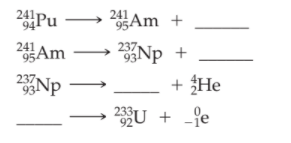×
Get Full Access to Introductory Chemistry - 5 Edition - Chapter 17 - Problem 65p
Get Full Access to Introductory Chemistry - 5 Edition - Chapter 17 - Problem 65p

×

# Fill in the blanks in the partial decay seriesISBN: 9780321910295 34

## Solution for problem 65P Chapter 17

Introductory Chemistry | 5th Edition

• Textbook Solutions
• 2901 Step-by-step solutions solved by professors and subject experts
• Get 24/7 help from StudySoup virtual teaching assistantsIntroductory Chemistry | 5th Edition

4 5 1 256 Reviews
14
2
Problem 65P

Fill in the blanks in the partial decay series.Step-by-Step Solution:
Step 1 of 3

Solution 65P:

Here, we are going to fill in the given blanks.

Step1:

Nuclear equations show the changes in the atomic numbers of the nuclides (the number of protons) and the changes in their mass numbers (the sum of the numbers of protons and neutrons). The following table gives the changes that occurs due to nuclear decay or emissions.In each of the equations in the partial decay series, the sum of the superscripts (mass numbers, A) for the reactants must be equal to the sum of the superscripts for the products. Similarly, the sum of the subscripts (atomic numbers, Z) for the reactants must be equal to the sum of the subscripts for the products.

Step2:Pu   →Am   +eAm   →Np   +HeNp  →Pa   +HePa  →U   +e

-------------------------------

Step 2 of 3

Step 3 of 3

##### ISBN: 9780321910295

The answer to “?Fill in the blanks in the partial decay series.” is broken down into a number of easy to follow steps, and 9 words. Introductory Chemistry was written by and is associated to the ISBN: 9780321910295. Since the solution to 65P from 17 chapter was answered, more than 524 students have viewed the full step-by-step answer. This textbook survival guide was created for the textbook: Introductory Chemistry, edition: 5. This full solution covers the following key subjects: blanks, decay, fill, partial, Series. This expansive textbook survival guide covers 19 chapters, and 2046 solutions. The full step-by-step solution to problem: 65P from chapter: 17 was answered by , our top Chemistry solution expert on 05/06/17, 06:45PM.

Unlock Textbook Solution